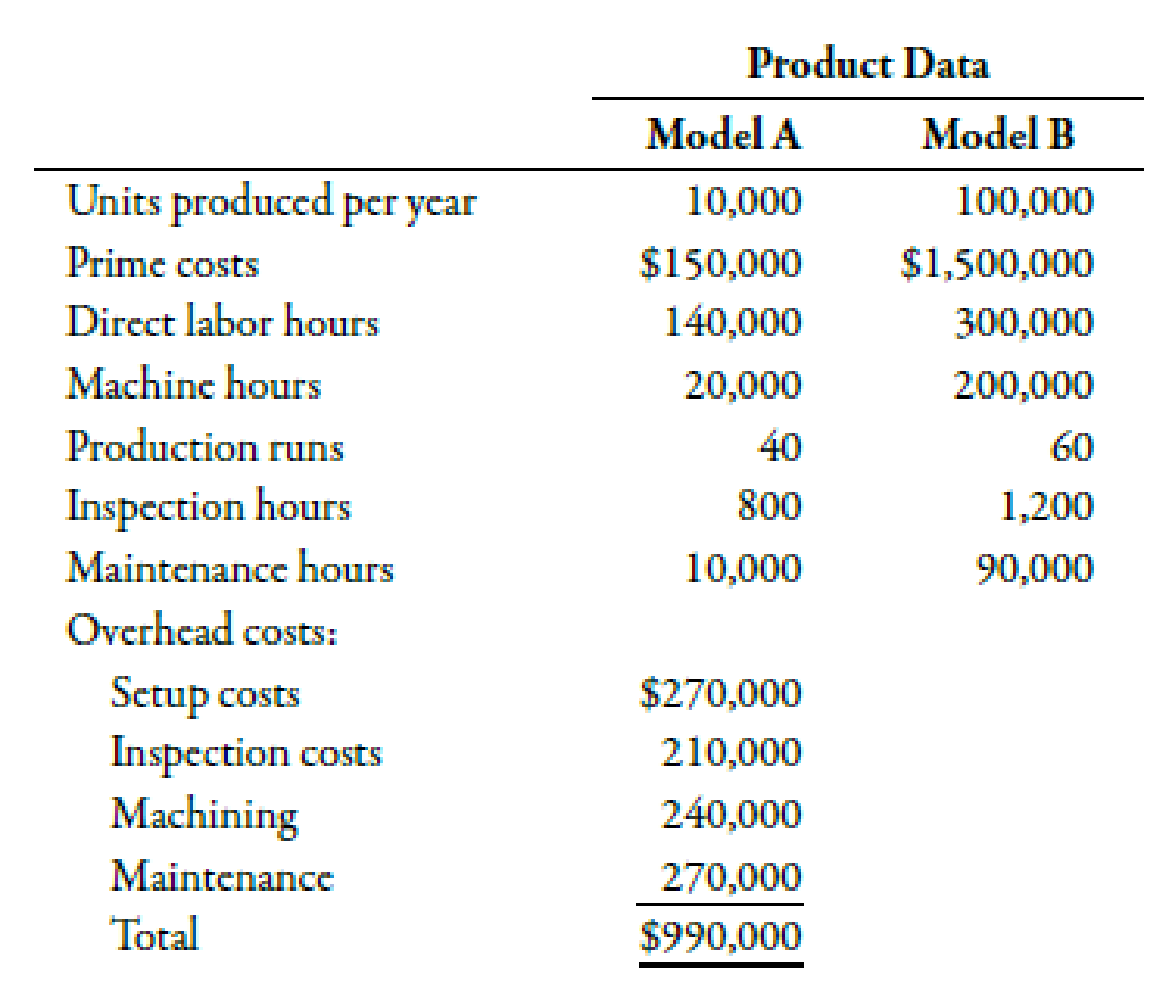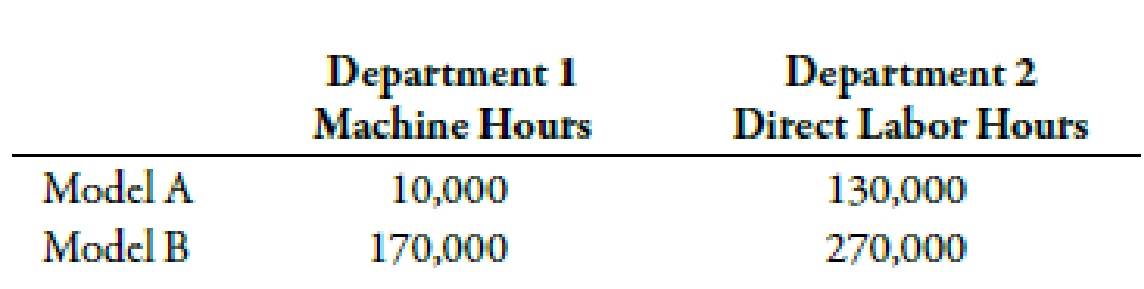Chapter 5, Problem 54P### Managerial Accounting: The Corners...

7th Edition
Maryanne M. Mowen + 2 others
ISBN: 9781337115773

#### Solutions

Chapter
Section### Managerial Accounting: The Corners...

7th Edition
Maryanne M. Mowen + 2 others
ISBN: 9781337115773
Textbook Problem
188 views

# Plantwide versus Departmental Rates, Product-Costing Accuracy: Activity-Based CostingRamsey Company produces speakers (Model A and Model B). Both products pass through two producing departments. Model A’s production is much more labor-intensive than that of Model B. Model B is also the more popular of the two speakers. The following data have been gathered for the two products:Required: 1. Compute the overhead cost per unit for each product by using a plantwide rate based on direct labor hours. (Note: Round to two decimal places.) 2. Compute the overhead cost per unit for each product by using ABC. (Note: Round rates and unit overhead cost to two decimal places.) 3. Suppose that Ramsey decides to use departmental overhead rates. There are two departments: Department 1 (machine intensive) with a rate of $3.50 per machine hour and Department 2 (labor intensive) with a rate of$0.90 per direct labor hour. The consumption of these two drivers is as follows:Compute the overhead cost per unit for each product by using departmental rates. (Note: Round to two decimal places.) 4. CONCEPTUAL CONNECTION Using the activity-based product costs as the standard, comment on the ability of departmental rates to improve the accuracy of product costing. Did the departmental rates do better than the plantwide rate?

1.

To determine

Compute overhead costs per unit with the help of plant wide rate.

Explanation

Activity Based Costing (ABC):

Activity based costing is a apportionment of costs that first considers the activity drivers that helps in the allocation of costs to various activities and then allocates costs to different cost objects by using the drivers.

Use the following formula to compute the overhead costs per unit for Model A:

Overhead Costs=Plantwide Rate1×Direct Labor HoursUnits Produced

Substitute $2.25 for plant wide rate and 140,000 direct labor hours and 10,000 units for units produced in the above formula. Overhead Costs=$2.25×140,00010,000units=$31.50 Therefore, an overhead cost per unit of Model A is$31.50.

Use the following formula to compute the overhead costs per unit for Model B:

Overhead Costs=Plantwide Rate1×Direct Labor HoursUnits Produced

Substitute \$2

2.

To determine

Calculate the overhead costs per unit with the help of ABC approach.

3.

To determine

Calculate the overhead costs per unit with the help of departmental rates.

4.

To determine

Discuss the ability of departmental rates to improve the accuracy of product costing. Also, identify that the departmental rates are better than the plant wide rate or not.

### Still sussing out bartleby?

Check out a sample textbook solution.

See a sample solution

#### The Solution to Your Study Problems

Bartleby provides explanations to thousands of textbook problems written by our experts, many with advanced degrees!

Get Started

#### Why is productivity important?

Principles of Microeconomics (MindTap Course List)

#### Some income from capital is taxed twice. Explain.

Brief Principles of Macroeconomics (MindTap Course List)

#### INTEREST RATE PREMIUMS A 5-year Treasury bond has a 5.2% yield. A 10-year Treasury bond yields 6.4%, and a 10-y...

Fundamentals of Financial Management, Concise Edition (with Thomson ONE - Business School Edition, 1 term (6 months) Printed Access Card) (MindTap Course List)